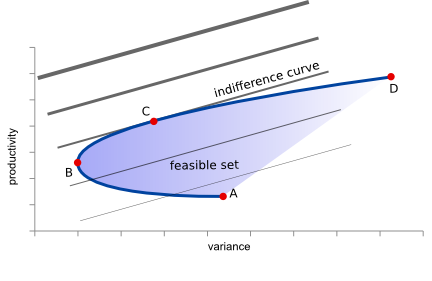This is an old revision of the document!

PortOpt [Portfolio Optimizer] is an open-source (LGPLed) C++ program (with Python binding) implementing the Markowitz(1952) mean-variance model with agent's linear indifference curves toward risk in order to find the optimal assets portfolio under risk.

You have to provide PortOpt (in text files or - if you use the api- using your own code) the variance/covariance matrix of the assets, their average returns and the agent risk preference.

It returns the vector of assets' shares that compose the optimal portfolio.

In order to minimise the variance it internally uses QuadProg++, a library that implement the algorithm of Goldfarb and Idnani for the solution of a (convex) Quadratic Programming problem by means of an active-set dual method.

Windows executable (command line tool!), linux (x64) executable and source code (for C++/Python) are available from SourceForge.

## Bugs and support

If you find a bug, request a feature or need support open a ticket or discuss it in the SourceForge pages.

## Theorical Background

In portfolio theory agents attempts to maximise portfolio expected return for a given amount of portfolio risk, or equivalently to minimise risk for a given level of expected return.The portfolio management can be portrayed graphically as in the above Figure, where the feasible set of variance-profitability combinations in enclosed by the blue curve and the B-D segment represents the efficient frontier, where no variance can be lowered at productivity's price or equivalently no productivity can be increased at price of increasing variance.

In order to simplify computations, forest managers are assumed to have risk aversion with simple linear preferences, that is they are willing to trade off variance with productivity proportionally. In such case the indifference curves can be drawn like a bundle of straight lines having equation $prod = \alpha * var + \beta$, where $\alpha$ is the linear risk aversion coefficient and both $prod$ and $var$ refer to the overall portfolio's productivity and variance.

Point $B$ represents the point having the lowest possible portfolio variance. Agents with $\alpha$ risk aversion will choose however the tangent point $C$ that can be obtained by solving the following quadratic problem:

\begin{equation} \begin{array}{rrrll} \max_{x_i, \beta} & Y & = & \beta & \\ s.t. & & & & \\ & x_i & \geqslant & 0 & \forall i\\ & \sum_i x_i & = & 1 & \\ & \sum_i {x_i p_i} & = & \alpha \sum_i { \sum_j { x_i x_j \sigma_{i,j}}} + \beta & \\ \end{array} \label{eq:optimisation_problem1} \end{equation}

that by substitution become:

\begin{equation} \begin{array}{rrrll} \min_{x_i} & Y & = & \alpha \sum_i { \sum_j { x_i x_j \sigma_{i,j}}} - \sum_i {x_i p_i} & \\ s.t. & & & & \\ & x_i & \geqslant & 0 & \forall i\\ & \sum_i x_i & = & 1 & \\ \end{array} \label{eq:optimisation_problem2} \end{equation}

where $x_i$ is the share of the asset $i$, $p_i$ is its productivity and hence $\sum_i {x_i p_i}$ is the overall portfolio productivity and $\sum_i { \sum_j { x_i x_j \sigma_{i,j}}}$ its variance.

As the only quadratic term arises when $i=j$ and $\sigma_{i,j}$ being the variance is always positive, the problem is convex and hence easily numerically solved.

## Compilation (not needed if using a pre-compiled version)

#### Option 1 - as a stand-alone program

g++ -std=c++0x -O -o portopt_executable QuadProg++.cpp Array.cpp anyoption.cpp portopt.cpp  main.cpp

#### Option 2 - as a library to be used in your C/C++ program

g++ -fPIC -std=c++0x -O -c QuadProg++.cpp Array.cpp anyoption.cpp portopt.cpp main.cpp
g++ -std=c++0x -O -shared -Wl,-soname,portopt.so -o portopt.so QuadProg++.o Array.o anyoption.o portopt.o

g++ -std=c++0x -O -o portopt_executable main.o -Wl,-rpath,'\$ORIGIN' -L . portopt.so
./portopt_executable

#### Option 3 - as a lib to be used in python, using swig

swig -c++ -python portopt.i
g++ -fPIC -std=c++0x -O -c QuadProg++.cpp Array.cpp anyoption.cpp portopt.cpp portopt_wrap.cxx main.cpp -I/usr/include/python2.7 -I/usr/lib/python2.7
g++ -std=c++0x -O -shared -Wl,-soname,_portopt.so -o _portopt.so QuadProg++.o Array.o anyoption.o portopt.o portopt_wrap.o

(then please refer to the python example for usage)

## Usage

##### Linux
./portopt [options]
##### Windows
portopt.exe [options]

(from a DOS prompt: (a) START→run→“cmd”; (b) cd \path\to\portopt )

##### As a lib from C++:

Call:

double solveport (const vector< vector <double> > &VAR, const vector<double> &MEANS, const double &alpha, vector<double> &x_h, int &errorcode)
##### As a lib ising Python:
import portopt
results = portopt.solveport(var,means,alpha)

#### Options

  -h  --help                                    Prints this help
-v  --var-file [input_var_file_name]         Input file containing the variance/covariance matrix (relative path)
-m  --means-file [input_means_file_name]     Input file containing the means vector (relative path)
-a  --alpha [alpha_coefficient]              Coefficient between production and risk in the linear indifference curves
-f  --field-delimiter [field_delimiter]      Character to use as field delimiter (default: ';')
-s  --decimal-separator [decimal-separator]  Character to use as decimal delimiter (default: '.')''  
• Higher the alpha, lower the agent risk aversion;
• Set a negative alpha to retrieve the portfolio with the lowest possible variance;
• Set alpha to zero to retrieve the portfolio with the highest mean, indipendently from variance (solution not guaranteed to be unique);
• Assets shares are returned in the x_h vector, eventual error code (0: all fine, 1: input data error, 2: problem has no solutions, 3: internal solver error) in the errorcode parameter.

PortOpt is free software: you can redistribute it and/or modify it under the terms of the GNU Lesser General Public License as published by the Free Software Foundation, either version 3 of the License, or (at your option) any later version.

PortOpt is distributed in the hope that it will be useful, but WITHOUT ANY WARRANTY; without even the implied warranty of MERCHANTABILITY or FITNESS FOR A PARTICULAR PURPOSE. See the GNU General Public License for more details.

You should have received a copy of the GNU Lesser General Public License along with PortOpt. If not, see http://www.gnu.org/licenses.

## Acknowledgements

This work was supported by:

• a grant overseen by Office National des Forêts through the Forêts pour Demain International Teaching and Research Chair;
• the French National Research Agency through the Laboratory of Excellence ARBRE, a part of the “Investissements d'Avenir” Program (ANR 11 – LABX-0002-01).